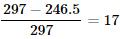Courses

# Competition Level Test: Structure of Atom- 1

## 10 Questions MCQ Test Chemistry for JEE | Competition Level Test: Structure of Atom- 1

Description
This mock test of Competition Level Test: Structure of Atom- 1 for JEE helps you for every JEE entrance exam. This contains 10 Multiple Choice Questions for JEE Competition Level Test: Structure of Atom- 1 (mcq) to study with solutions a complete question bank. The solved questions answers in this Competition Level Test: Structure of Atom- 1 quiz give you a good mix of easy questions and tough questions. JEE students definitely take this Competition Level Test: Structure of Atom- 1 exercise for a better result in the exam. You can find other Competition Level Test: Structure of Atom- 1 extra questions, long questions & short questions for JEE on EduRev as well by searching above.
QUESTION: 1

Solution:

Alpha rays as the least penetrating, followed by beta rays, followed by gamma rays as the most penetrating.

QUESTION: 2

### For a hypothetical H like atom which follows Bohr's model, some spectral lines were observed as shown. If it is known that line 'E' belongs to the visible region, then the lines possibly belonging to ultra violet region will be (n1 is not necessarily ground state)            [Assume for this atom, no spectral series shows overlaps with other series in the emmission spectrum]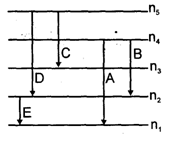Solution:

In the given figure if line 'E' is in visible region then line belonging to ultraviolet region will have more energy than 'E' i.e. line A

QUESTION: 3

### The number of photons emitted in 10 hours by a 60W sodium lamp (λ, of photon = 6000 Å)                [Take he = 12400 EvÅ, h = Planck's constant, c = speed of light]

Solution: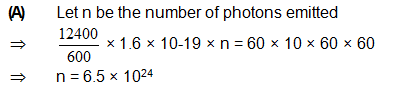QUESTION: 4

Radius of 3rd orbit of Li2+ ion is ‘x’ cm then de-broglie wavelength of electrons in the 1st orbit is

Solution: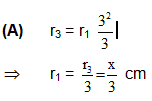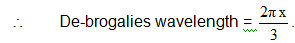QUESTION: 5

Ratio of frequency of revolution of electron in the second state of He revolution of electron in the second state HeΘ and second state of hydrogen is

Solution: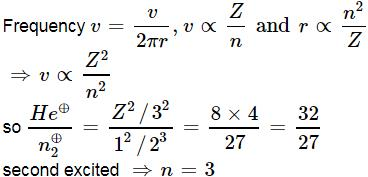QUESTION: 6

In any subshell, the maximum number of electrons having same value of spin quantum number is:

Solution:

Maximum number of electron having same spin quantum number is equal to the number of orbitals =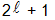QUESTION: 7

A proton accelerated from rest through a potential difference of 'V' volts has a wavelength λ associated with it. An alpha particle in order to have the same wavelength must be accelerated from rest through a potential difference of

Solution: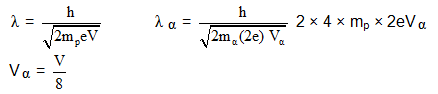QUESTION: 8

Consider the ground state of Cr atom (Z = 24). The numbers of electrons with the azimuthal quantum numbers,  = 1 and 2 are, respectively:

Solution:

Sol.    = 1 p and  = 2 for d.

Now Cr has configuration

1s2 2s2 2p6 3s2 3p6 3d5 4s1

Hence there are 12, p-electrons and 5, d-electrons

QUESTION: 9

Number of electrons having l + m value equal to zero in 26Fe may be

Solution: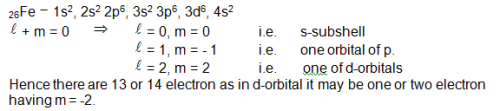QUESTION: 10

A photon of 4000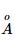is used to break the iodine molecule, then the % of energy converted to the K.E. of iodine atoms if bond dissociation energy of I2 molecule is 246.5 kJ/mol is:

Solution:

Energy of one photon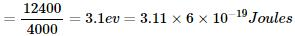Energy supplied by one mole photon in KJ/mole = 3.11×6×10−19×6×1023×10−3=297KJ/mole
% of energy converted to K.E.=